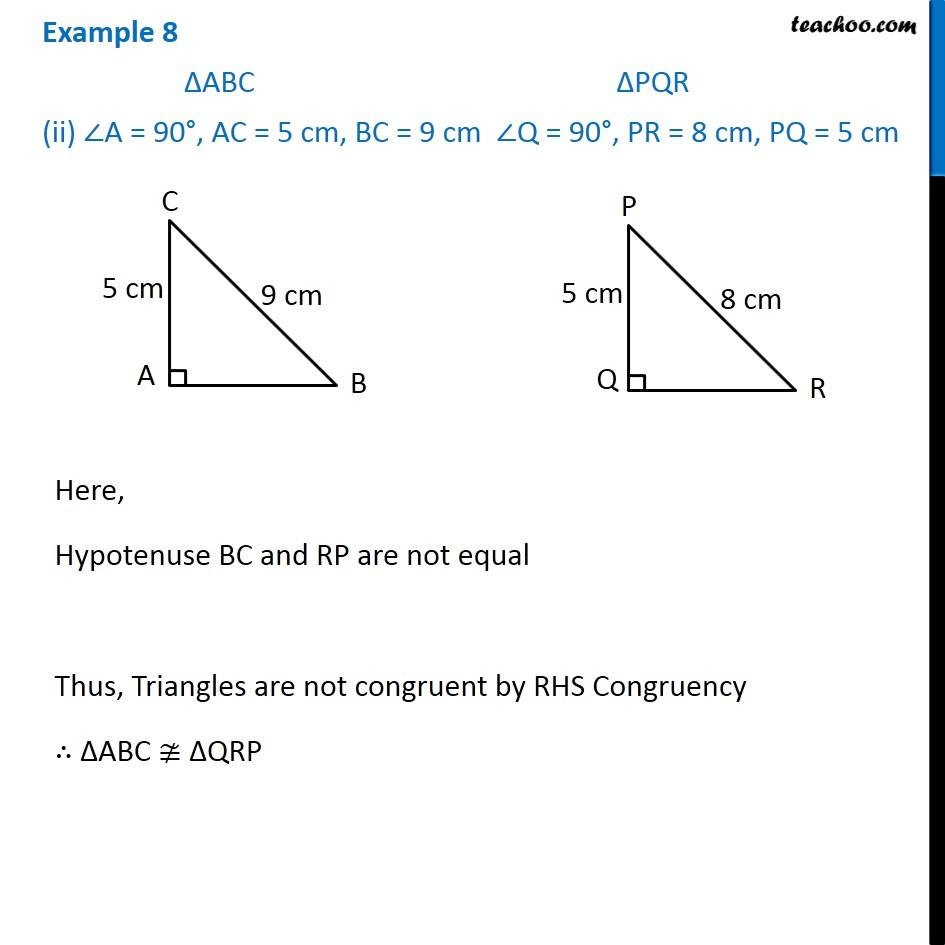Examples

Chapter 7 Class 7 Congruence of Triangles
Serial order wise### Transcript

Example 8 ∆ABC ∆PQR (ii) ∠A = 90°, AC = 5 cm, BC = 9 cm ∠Q = 90°, PR = 8 cm, PQ = 5 cm Here, Hypotenuse BC and RP are not equal Thus, Triangles are not congruent by RHS Congruency ∴ ∆ABC ≇ ∆QRP